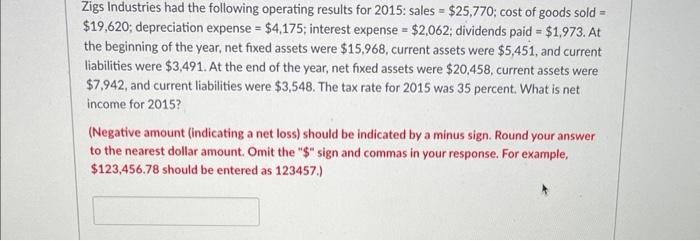# (Solved): Zigs Industries had the following operating results for 2015 : sales \( =\\$ 25,770 \); cost of good ...Zigs Industries had the following operating results for 2015 : sales \( =\\$ 25,770 \); cost of goods sold \( = \) \( \\$ 19,620 \); depreciation expense \( =\\$ 4,175 \); interest expense \( =\\$ 2,062 \); dividends paid \( =\\$ 1,973 \). At the beginning of the year, net fixed assets were \( \\$ 15,968 \), current assets were \( \\$ 5,451 \), and current liabilities were \( \\$ 3,491 \). At the end of the year, net fixed assets were \( \\$ 20.458 \), current assets were \( \\$ 7,942 \), and current liabilities were \( \\$ 3,548 \). The tax rate for 2015 was 35 percent. What is net income for 2015? (Negative amount (indicating a net loss) should be indicated by a minus sign. Round your answer to the nearest dollar amount. Omit the " \( \\$ \) " sign and commas in your response. For example, \( \\$ 123,456.78 \) should be entered as \( 123457 .) \)

We have an Answer from Expert